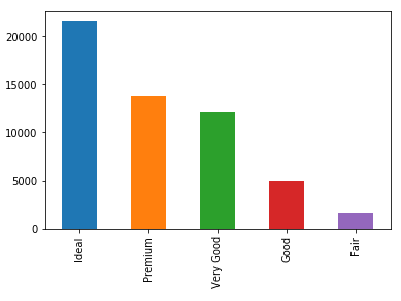﻿ Pandas Practice Set-1: Create a bar plot of the 'value_counts' for the 'cut' series of diamonds DataFrame - w3resource# Pandas Practice Set-1: Create a bar plot of the 'value_counts' for the 'cut' series of diamonds DataFrame

## Pandas Practice Set-1: Exercise-38 with Solution

Write a Pandas program to create a bar plot of the 'value_counts' for the 'cut' series of diamonds DataFrame.

Sample Solution:

Python Code:

``````import pandas as pd
print("Original Dataframe:")
print("\nBar plot of the 'value_counts' for the ‘cut’ series of diamonds DataFrame:")
diamonds.cut.value_counts().plot(kind='bar')
``````

Sample Output:

```Original Dataframe:
carat      cut color clarity  depth  table  price     x     y     z
0   0.23    Ideal     E     SI2   61.5   55.0    326  3.95  3.98  2.43
1   0.21  Premium     E     SI1   59.8   61.0    326  3.89  3.84  2.31
2   0.23     Good     E     VS1   56.9   65.0    327  4.05  4.07  2.31
3   0.29  Premium     I     VS2   62.4   58.0    334  4.20  4.23  2.63
4   0.31     Good     J     SI2   63.3   58.0    335  4.34  4.35  2.75

Bar plot of the 'value_counts' for the ‘cut’ series of diamonds DataFrame:```

Python Code Editor:

Have another way to solve this solution? Contribute your code (and comments) through Disqus.

What is the difficulty level of this exercise?

﻿

## Python: Tips of the Day

Python: Debug With the Print() Function

```>>> for i in range(5):
...     print(i, end=', ' if i < 4 else '\n')
...
0, 1, 2, 3, 4
>>> for i in range(5):
...     print(f'{i} & {i*i}', end=', ' if i < 4 else '\n')
...
0 & 0, 1 & 1, 2 & 4, 3 & 9, 4 & 16
```